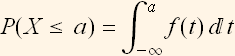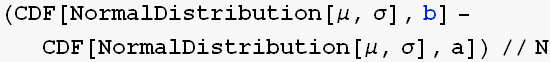Using Mathematica to study basic probability

by Nasser Abbasi, september 30, 2007

Basic relations. Let f(x) be a PDF for some continuous random variable.  Then the following is trueAs can be verified as follows (using Normal distribution as an example) by evaluating the above integral and see if it the same as CDF(X=a)

In:=Out=Now find F (a), it should be the same as aboveAnother important relation is probability of X being in some range. This is the same as the area under the curve of f(x) between the 2 points:The above is found as followsNow find F (b)-F(a), it should be the same as abovelets try to see how to find probabilty of X be in some range when X is discrete. Assume X is a discrete random variable, say a Binomial. We need to do the same as above. Now we can not use Integrate, but need to us SUMLet find P (X < 3) in the above. Now instead of integration, we use sum, we want to add the area under the PDF from 0 to 3. But the width is 1 for each interval. So we just sum the y values.Verify by checking the CDF at 3, it should be the same as aboveTo show that probability mass function adds to one. Say we have binomial distributionCreated by Wolfram Mathematica 6.0 for Students - Personal Use Only  (29 December 2007)